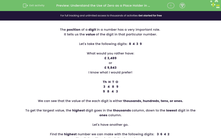# Understand the Use of Zero as a Place Holder in 4-Digit Numbers

In this worksheet, students will find the value of digits in numbers, including the use of zero as a place holder.Key stage:  KS 2

Curriculum topic:   Number: Number and Place Value

Curriculum subtopic:   Recognise Thousands, Hundreds, Tens and Ones

Difficulty level:#### Worksheet Overview

The position of a digit in a number has a very important role.

It tells us the value of the digit in that particular number.

Let's take the following digits:  8  4  3  9

What would you rather have:

£ 3,489

or

£ 9,843

I know what I would prefer!

Th  H  T  O

3   4   8   9

9   8   4   3

We can see that the value of the each digit is either thousands, hundreds, tens, or ones.

To get the largest value, the highest digit goes in the thousands column, down to the lowest digit in the ones column.

Let's have another go.

Find the highest number we can make with the following digits:   3  6  4  2

The digits will go in the following columns:

Th  H  T  O

6   4   3   2

The highest digit goes in the thousands column down to the lowest in the ones column.

In this number, the digit has a value of 6,000

In this number, the 4 digit has a value of 400

In this number, the digit has a value of 30

In this number, the 2  digit has a value of 2Try some questions for yourself - good luck!

### What is EdPlace?

We're your National Curriculum aligned online education content provider helping each child succeed in English, maths and science from year 1 to GCSE. With an EdPlace account you’ll be able to track and measure progress, helping each child achieve their best. We build confidence and attainment by personalising each child’s learning at a level that suits them.

Get started